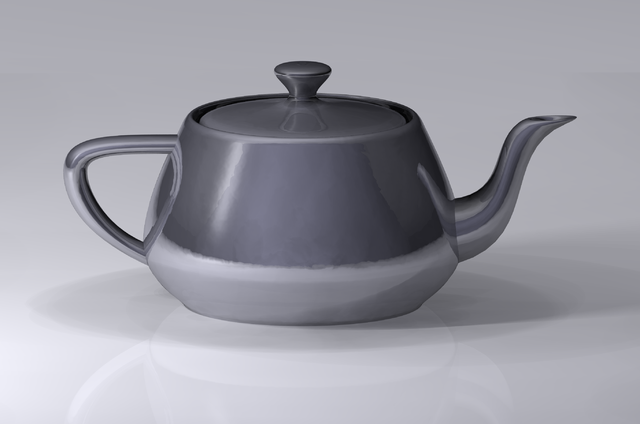# R - Histogram

How to make histogram in R.

typical plots with vertical bars are not histograms. See barplot or plot(*, type = “h”) for such bar plots

## Package

### Graphics

``````data = c(78 ,79 ,84 ,85 ,85 ,86 ,87 ,89 ,89 ,90 ,90 ,92 ,92 ,93 ,95 ,95 ,96 ,97 ,97 ,98)
hist(data)```
```

You can use the layout function in order to have more than one histogram by page.

Example:

``````# 4 histograms in a 2x2 matrix
layout(matrix(c(1,2,3,4), 2, 2, byrow = TRUE))```
```

### Ggplot

See Ggplot - Histogram (geom_histogram, geom_freqpoly): Histogram:

• Geom: bar
• Stat: bin
• Scale: linear
• Coordinate system: Cartesian

Recommended PagesData Visualisation - Histogram (Frequency distribution)

A histogram is a type of graph generally used to visualize a distribution An histogram is also known as a frequency distribution. Histograms can reveal information not captured by summary statistics...R - Density

The density function This function computes kernel density estimates. The algorithm used in density.default disperses the mass of the empirical distribution function over a regular grid of at least 512...